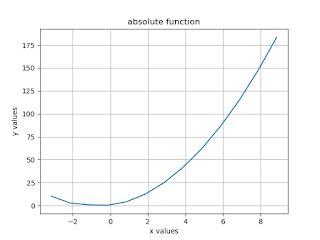## Absolute function in Python: In this section, We discuss the Program to Plotting absolute function using various Python Libraries. The absolute function can be defined using the following syntax.

Syntax - abs(number)

Python abs()- It is an inbuilt function that returns the absolute value of the given number. In the case of a complex number, the abs() method returns its magnitude.  Python abs() function takes only one argument. It can be the integer, a floating-point number, or a complex number. When an argument is an integer or floating-point number, abs() returns the absolute value in integer or float.  If it is a complex number, abs() returns only the magnitude part, and that can also be a floating-point number.

integer
floating number
complex number

## abs() Returns value: The following value returns by an absolute function.

Integer, Long – it returns absolute value.
Float – it returns absolute value.
Complex – returns its magnitude
It returns the absolute value in the decimal system even if numbers are defined in binary, octal, hexadecimal or exponential form.
Now take a Look at the example of the absolute function in the case of integers, floating-point numbers, a complex numbers.

## How do Python abs() function work:

The abs() function works on the following way on the numbers:
1. Integers, for example 5, -5, 1, etc.
2. Floating point numbers, for example, 5.55, -1.34, etc
3. Complex numbers, for example 2+4j, 4+5j etc.

Now take a look at how to find out the absolute value of an integer, floating-point number and complex number by using the Python code.

1. an integer:
```integer = -10print('Absolute value of integer is:',abs(integer))
```
Output: Absolute value of the integer is: 10

2. floating-point number:
`floating = -40.44print('Absolute value of floating number is:',abs(floating))`
`Output:Absolute value of floating number is:40.44`
3. Complex Number:
```complex = (2 - 4j)
print('Magnitude of 2 - 4j is:',abs(complex))```
```Output:Magnitude of 2 - 4j is: 4.47213595499958
```
Now take a look at how to plot absolute function in Python Programming using numpy and matplotlib library.

Python Code:
import numpy as np
from matplotlib import pyplot as plt
x = np.arange(-
3.14,3*3.14)
a =
2*x
b = x*
3
y = np.abs(a*x+b)
plt.plot(x,y)
plt.xlabel(
'x values')
plt.ylabel(
'y values')
plt.title(
'absolute function')
plt.grid(
True
)
plt.show()

Output:Program to Plotting absolute funcction
Summary: In this section, We discussed the absolute function in Python with code and examples. It a function that returns the absolute value of a given function. So during this discussed the definition of the absolute function, the syntax for the absolute function and parameters are used in the absolute function.

You can also enter an integer, a floating number and a complex number from the user instead of declaring in the code as we show in the example of the absolute function.

Then Discussed the Code of the absolute function in Python Programming using Numpy and Matplotlib library. In the last, We discussed the way to plot an absolute function in Python Programming.